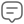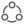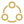Return on investment, or ROI, is one of the most popular terms that every trader must know. In this article, Coin98 Insights will provide you with everything you need to know about Return on Investment.

## What is ROI (Return On Investment)?

ROI (Return on investment) is a measurement parameter to evaluate the effectiveness of a trade. It is often represented in the form of a percentage or a ratio. Traders can use ROI to simply and quickly determine whether a trade is profitable or not.

A positive ROI indicates that the trade is producing returns, while a negative one suggests losses. A good trader would likely maintain a high ROI and mitigate losses by closing the position if a trade has an overwhelming negative ROI.

ROI is an extremely useful tool to track crypto trades, especially the ones with leverage. A lot of traders use ROI as an indicator of when to take profits and when to stop losses.

For example, an ROI of 100% means your trade has doubled in value, and it should be closed to maintain profitability. On the other hand, an ROI of -20% may be incredibly alarming, especially for a leverage position - with a 5x leverage, this would already result in liquidation.

## How to calculate ROI

ROI can be calculated by using the following formula:

ROI = Net profit / Cost of investment

In which:

Net profit = Current investment value - Initial investment value

You can also add a multiplication of 100 at the end of the formula to get the percentage value.

Let’s put it in an example. Say that Alice invested in Bitcoin (BTC) with \$1,000 at the price of \$40,000. If the price of Bitcoin rises to \$44,000, Alice’s investment is now worth \$1,100. The ROI of her trade can be calculated as:

(1,100 - 1,000) / 1,000 = 0.1

If you want to calculate it in the percentage form, then multiply the above result with 100:

0.1 * 100 = 10 (%)

An ROI of 10% indicates that Alice is earning a profit of 10% from her investment, which equals to \$100. Now let’s try to complicate the problem by adding a 10x leverage into Alice’s investment.

The value of Alice’s investment now becomes \$10,000. After the increase in Bitcoin’s price, her trade is worth \$11,000. The ROI of her trade with a 10x leverage can be calculated as:

(11,000 - 10,000) / 1,000 = 1

If you want to calculate it in the percentage form, then multiply the above result with 100:

1 * 100 = 100 (%)

As you can see, with a 10x leverage, Alice’s investment has increased 10 times in profit: from 10% to 100%. This makes total sense and should give you a comprehensive overview of how to calculate ROI.

For those who just want a shortcut, you can use this online tool to calculate ROI: www.calculator.net/roi-calculator.html

## How to determine a good ROI

A good ROI varies between traders based on one’s preferences and investing strategy. For instance, some traders want to take profits with an ROI of 20 to 50%, while others would want to close their positions only with an ROI of 100% or higher. Therefore, it is difficult to determine a good ROI: this depends on you individually.

Nevertheless, there is one certain thing when it comes to determining a good ROI: the ROI has to be positive. As mentioned above, a positive ROI means a profitable trade, while a negative one indicates losses. Therefore, it can be safely said that a positive ROI is a good ROI.

## Limitations of ROI

ROI is a useful tool for investors to help them improve their trading efficiency. It can be calculated easily and quickly, giving traders the opportunity to make decisions on their trades immediately. Nevertheless, as a simple indicator, ROI has a few limitations:

• It completely disregards the value of time. The duration of a trade is not considered in ROI. For that reason, a high ROI does not necessarily mean a good trade: If a 20% ROI can be reached within a short period of time, it indicates a decent trade. For longer durations like years, it might not be as efficient.
• Other factors may affect ROI. This is especially true for low-cap assets in DeFi. In some cases, your ROI may be pre-calculated to be 100%, but your realized profits may not be able to maintain such a number. Due to other factors like price slippage, lack of liquidity,... the actual profits can be much smaller than the calculated ROI.
• For long-term investments, inflation also needs to be considered. In crypto, this might not be as relevant. Nevertheless, in traditional financial markets, a 10-year investment can be heavily impacted by inflation, hence reducing the actual profits. ROI does not acknowledge the influence of inflation.

### Return On Investment (ROI) vs. Rate Of Return (ROR)

Return On Investment (ROI) is closely similar to Rate of Return (ROR). In fact, the only difference is that Rate Of Return takes the matter of time into account, which is often calculated on a yearly basis.

As a result, ROR may be more efficient than ROI as a measurement parameter for investment since time is also an important factor, as mentioned above. In some cases, ROR can be equal to ROI.

### Does crypto have the highest ROI?

Even though we cannot say or guarantee that the crypto market has the highest ROI compared to others, it has been able to maintain an incredible ROI rate. This is an infographic made by our team, showing how profitable investing in Bitcoin on a monthly basis is.

### Do high ROIs mean high risk?

“High risk, high reward” is an exemplary quote for this question. Even though high-risk investments usually return high profits, it does not necessarily mean vice versa. Some of the safest investment choices can bring even higher profits than the risky ones.

## Conclusion

Return on investment (ROI) is a measurement parameter to evaluate the effectiveness of a trade. It is often represented in the form of a percentage or a ratio. Traders can use ROI to simply and quickly determine whether a trade is profitable or not.

ROI can be calculated by using the following formula: ROI = Net profit / Cost of investment. You can also add a multiplication of 100 at the end of the formula to get the percentage value.

ROI is a useful tool for investors to help them improve their trading efficiency. It can be calculated easily and quickly, giving traders the opportunity to make decisions on their trades immediately. Nevertheless, as a simple indicator, ROI has a few limitations. Other methods, like Rate Of Return (ROR), can also be used as an alternative for better efficiency in some cases.Comment

You need toorto comment